FX:EURUSD   Euro / U.S. Dollar
5599 views
5599
1. Price crosses moving average & Rejection
2. Enter short on signal
3. 1 Min Time Frame= 1 min expiry +

* Dont rely on signals / Trade what you see / Price action
*Use ma's as support = rejection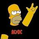// http://www.vdubus.co.uk/
strategy(title='Vdub FX SniperVX3 / Strategy v3', shorttitle='Vdub_FX_SniperVX3_Strategy', overlay=true, pyramiding=0, initial_capital=1000, currency=currency.USD)

//Candle body resistance Channel-----------------------------//
len = 34
src = input(close, title="Candle body resistance Channel")
out = sma(src, len)
last8h = highest(close, 13)
lastl8 = lowest(close, 13)
bearish = cross(close,out) == 1 and falling(close, 1)
bullish = cross(close,out) == 1 and rising(close, 1)
channel2=input(false, title="Bar Channel On/Off")
ul2=plot(channel2?last8h:last8h==nz(last8h)?last8h:na, color=black, linewidth=1, style=linebr, title="Candle body resistance level top", offset=0)
ll2=plot(channel2?lastl8:lastl8==nz(lastl8)?lastl8:na, color=black, linewidth=1, style=linebr, title="Candle body resistance level bottom", offset=0)
//fill(ul2, ll2, color=black, transp=95, title="Candle body resistance Channel")

//-----------------Support and Resistance
RST = input(title='Support / Resistance length:', type=integer, defval=10)
RSTT = valuewhen(high >= highest(high, RST), high, 0)
RSTB = valuewhen(low <= lowest(low, RST), low, 0)
RT2 = plot(RSTT, color=RSTT != RSTT ? na : red, linewidth=1, offset=+0)
RB2 = plot(RSTB, color=RSTB != RSTB ? na : green, linewidth=1, offset=0)

//--------------------Trend colour ema------------------------------------------------//
src0 = close, len0 = input(13, minval=1, title="EMA 1")
ema0 = ema(src0, len0)
direction = rising(ema0, 2) ? +1 : falling(ema0, 2) ? -1 : 0
plot_color = direction > 0 ? lime: direction < 0 ? red : na
plot(ema0, title="EMA", style=line, linewidth=1, color = plot_color)

//-------------------- ema 2------------------------------------------------//
src02 = close, len02 = input(21, minval=1, title="EMA 2")
ema02 = ema(src02, len02)
direction2 = rising(ema02, 2) ? +1 : falling(ema02, 2) ? -1 : 0
plot_color2 = direction2 > 0 ? lime: direction2 < 0 ? red : na
plot(ema02, title="EMA Signal 2", style=line, linewidth=1, color = plot_color2)

//=============Hull MA//
show_hma = input(false, title="Display Hull MA Set:")
hma_src = input(close, title="Hull MA's Source:")
hma_base_length = input(8, minval=1, title="Hull MA's Base Length:")
hma_length_scalar = input(5, minval=0, title="Hull MA's Length Scalar:")
hullma(src, length)=>wma(2*wma(src, length/2)-wma(src, length), round(sqrt(length)))
plot(not show_hma ? na : hullma(hma_src, hma_base_length+hma_length_scalar*6), color=black, linewidth=2, title="Hull MA")

//============ signal Generator ==================================//
Piriod=input('720')
ch1 = security(tickerid, Piriod, open)
ch2 = security(tickerid, Piriod, close)
longCondition = crossover(security(tickerid, Piriod, close),security(tickerid, Piriod, open))
if (longCondition)
strategy.entry("BUY", strategy.long)
shortCondition = crossunder(security(tickerid, Piriod, close),security(tickerid, Piriod, open))
if (shortCondition)

Sorry V this script is from another strategy of yours,started on this platform a week ago,saw this ,now i am not an expert like you,but I like to analise.
Wat Ive done is eliminate everything except the arrows,they give many false signals ,but what ive done is place rsi underneath with 17 period ,when arrow appears check rsi,if ob or os then arrow is more reliable but I still have to see what expiry time is best 3mins 5mins 10mins dont know will have to do more tests.
I hope im not sounding stupid.
Please forgive me people,im a newbee in all this.
Reply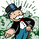Hey @vdubus is there any way to create text alerts for these buy/sell signals? I've been at for about 8 hours and I can't figure it out. Thanks!
Reply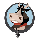ArmoredBull
wondering the same thing. Did anyone figure this out?
Reply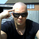Hi Vdubus what is Moving average format ??? And link for download gonna be great.
God bless you.
Reply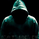steven.kompakt
ye I don't know whats going on there this here is the original link
Reply